Wang, Z., Wang, W., Ma, D., Guo, X., Huan, J., and Cheng, L. (2020). "Coupling model of fuzzy soft set and Bayesian method to forecast internal defects of ancient wooden structures based on nondestructive test," BioRes. 15(1), 1134-1153.

#### Abstract

In order to improve the detection precision of internal defect in the ancient wooden structures, defect simulation tests on pine and elm commonly used in ancient buildings were performed by using stress wave detection and drilling resistance detection. Based on detection data, three typical evaluation criteria, which are the information entropy, the correlation coefficient, and residual sum of squares, were selected as a priori information. Combining with the expert’s fuzzy evaluation value, Bayesian formula was used to modify the prior information to determine the weight coefficients of the two detection methods, and a combined prediction model was established. The results show that the combination of subjectivity and objectivity enables the revised weights to more reasonably and accurately reflect the relative importance of each detection method in prediction evaluation, which reduces the forecasting error. Specifically speaking, the mean error of the combined model was reduced by 49.8% and 59.8%, respectively, compared with stress wave detection and drilling resistance detection. Moreover, the five error indicators of this combined forecasting model are the smallest in all methods, indicating the proposed method has a better forecasting effect. It provides an effective application tool for the practice of forecasting the internal defects of wooden components in ancient buildings.

Coupling Model of Fuzzy Soft Set and Bayesian Method to Forecast Internal Defects of Ancient Wooden Structures Based on Nondestructive Test

Ziyi Wang,a,c,d Wei Wang,b,c,d,* Donghui Ma,b,c,d Xiaodong Guo,b,c,d Junhong Huan,a,c,d and Liting Cheng a

In order to improve the detection precision of internal defect in the ancient wooden structures, defect simulation tests on pine and elm commonly used in ancient buildings were performed by using stress wave detection and drilling resistance detection. Based on detection data, three typical evaluation criteria, which are the information entropy, the correlation coefficient, and residual sum of squares, were selected as a priori information. Combining with the expert’s fuzzy evaluation value, Bayesian formula was used to modify the prior information to determine the weight coefficients of the two detection methods, and a combined prediction model was established. The results show that the combination of subjectivity and objectivity enables the revised weights to more reasonably and accurately reflect the relative importance of each detection method in prediction evaluation, which reduces the forecasting error. Specifically speaking, the mean error of the combined model was reduced by 49.8% and 59.8%, respectively, compared with stress wave detection and drilling resistance detection. Moreover, the five error indicators of this combined forecasting model are the smallest in all methods, indicating the proposed method has a better forecasting effect. It provides an effective application tool for the practice of forecasting the internal defects of wooden components in ancient buildings.

Keywords: Ancient building; Wooden structure; Internal defect; Non-destructive tests; Combined forecasting model; Fuzzy soft set; Bayesian method

Contact information: a: College of Architecture and Civil Engineering, Beijing University of Technology, Beijing 100124, China; b: College of Architecture and Urban Planning, Beijing University of Technology, Beijing 100124, China; c: Institute of Earthquake Resistance and Disaster Reduction, Beijing University of Technology, Beijing 100124, China; d: Key Science Research Base of Safety Assessment and Disaster Mitigation for Traditional Timber Structure (Beijing University of Technology), State Administration for Cultural Heritage, Beijing 100124, China; *Corresponding author: ieeww@bjut.edu.cn

INTRODUCTION

Compared with the masonry structure system of western ancient architecture, the most important feature of Chinese ancient architecture is that the building adopts a wooden structure system (Kim and Park 2017). However, timber components in ancient buildings often appear decayed, with insect attacks and hollow spaces (Fig. 1) after several hundred years or even thousands of years (Gao et al. 2018; Broda and Popescu 2019). As a result, the physical and mechanical properties are attenuated, which ultimately leads to the damage and the reduction of mechanical strength of wooden structure in ancient buildings (Calderoni et al. 2010; Qin and Yang 2016). For example, the Qinzhuang Dongyue Temple (Fig. 2a), the Jade Emperor Temple (Fig. 2b), and the Puming Temple (Fig. 2c), built in the Yuan Dynasty, are historical buildings in Shanxi Province, China. The Xia Jin Confucian Temple (Fig. 2d), built in the Song Dynasty, is a historical building in Shandong Province, China. Due to the lack of maintenance, long cracks, hollows, and insects appeared in the beams or columns, which eventually cause the roof to collapse.

The protection of wooden structures that have been used for hundreds of years has become a top priority in the maintenance and repair of ancient buildings in China. To ensure that the authenticity of the ancient structure is not destroyed and to accurately assess the health status of the wooden structure, it is necessary to obtain the internal damage information of wooden structures in the ancient buildings by nondestructive testing (Chen and Guo 2017; Voessing and Niederleithinger 2018).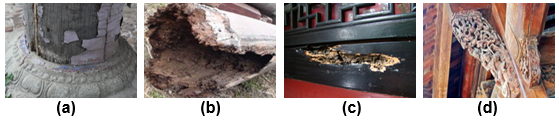Fig. 1. Damaged characteristics of (a) decay, (b) hollow, (c) insect attacks, (d) crack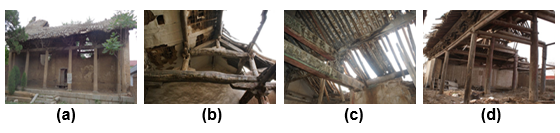Fig. 2. Destruction of wooden structures in (a) Dongyue Temple, (b) Jade Emperor Temple, (c) Puming Temple, (d) Xia Jin Confucian Temple

Many non-destructive testing methods have been developed, such as ultrasonic detection (Gatto et al. 2012; Vossing et al. 2018; Espinosa et al. 2019), X-ray detection (Pease et al. 2012; Franke et al. 2013; Riggio et al. 2015), pin impact tests (Huang et al. 2010; Wu et al. 2010), stress wave detection (Rust and Göcke 2000; Dackermann et al. 2014; Li et al. 2015), and drilling resistance detection (Isik and Li 2003; Kasal and Anthony 2004; Nowak et al. 2016). Pin impact tests, stress wave detection, and drilling resistance detection are the more common methods (An et al. 2008), but it is difficult to carry out a comprehensive and accurate assessment of the internal damage of wooden structures using only one method. To make up for the shortcomings of each method, different detection methods have been combined to analyze quantitatively and qualitatively the internal damage of wood (An et al. 2008; Ge et al. 2014; Wang and Allison 2008; Ouis and Zerizer 2006; Choi et al. 2007). Moreover, there are already several publications reporting on the determination of weights related to the combination of different nondestructive tests (Chang et al. 2016a,b; Dai et al. 2017; Chang et al. 2019; Wang et al. 2019). However, these combined forecasting models are often built solely based on specific evaluation criteria, and they only consider objective test data and ignore experts’ experience when determining the weights of the combined model. Therefore, the forecasting effect often has a large error relative to the actual situation. In fact, there are often large differences between the results of different detection methods. In the process of actual detection, experts often need to conduct field investigation based on experience, so as to subjectively and objectively give the forecasting results consistent with the actual situation. Based on the detection data and expert experience, one attempts to determine the weights of the combined model by using the experts’ evaluation fuzzy value to correct the prior information of nondestructive test, hoping to improve the precision of forecasting internal defects of wooden members.

Combined Forecasting Model of Internal Defect of Timber Components

When the stress wave detection method and drilling resistance detection method are used alone, their detection precision is usually relatively low, and it is difficult to obtain more comprehensive information on internal defects of wooden members (Wang et al. 2019). To be more specific, the detected image obtained by the stress wave detection method can intuitively show the decay distribution of the measured section, but the boundary of the image is very fuzzy and the detection results are not accurate enough. Meanwhile, the drilling resistance detection method can accurately reflect the material condition along the test direction, and it often requires multiple paths for detection, which will cause damage to the ancient wooden structure (Chang et al. 2016b; Wang et al. 2019). Therefore, a combined forecasting model is established in this work based on the stress wave detection and drilling resistance detection according to the characteristics of internal damage in wooden structure. Combined forecasting method can set up the coordination model based on a single detection method by combining the weight coefficients, which can achieve better forecasting effect. It is generally known that determining the combined weight coefficients is essential for combined forecasting method (Huang et al. 2015; Zhou and Wang 2009). At present, there are three types of methods, as follows:

1. According to evaluation criterion of a certain detection result, the optimization model is constructed to obtain the combined weighting coefficients (Chen and Hou 2003). However, this method is one-sided, and the forecasting effect evaluation indexes are various, which cannot improve forecasting effects.
2. The weight coefficients of the criterion are determined by using multi-criteria decision method, such as the entropy method. However, when the weight coefficients are determined by the entropy method, there is no guarantee that the combined forecasting value is better than the single method (Zhu and Yan 2004).
3. The information aggregation operator is used to determine the combined weight coefficients (Aggarwal 2015; Chen and Sheng 2005). This method relies on the knowledge background of each decision maker and has strong subjectivity.

In view of the above situations, according to the diversity of forecasting effect evaluation criteria, this paper selected three typical evaluation criteria—the information entropy (Shannon 1948; Lianxiao and Morimoto 2019), the correlation coefficient, and the residual sum of squares—to be a priori information. Combined weight coefficients of two detection methods are determined by using the unrestricted advantages of fuzzy soft set parameter tools and the advantage of Bayesian formula in modifying prior information (Sun 2014; Dong et al. 2018). The combination of subjectivity and objectivity can reduce the forecasting error, which provides an effective method for forecasting the internal damage of ancient wooden structures. The combined forecasting model of internal damage in wood under the condition t is as follows: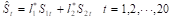(1)

where l*1 represents the corrected weight of stress wave detection value (S1t), l*2 represents the corrected weight of drilling resistance detection value (S2t), l*1+l*2=1.

Combinatorial Coefficients Based on Fuzzy Soft Set and Bayesian Method

Determine evaluation criteria

The detection error value refers to the difference between the actual value and the detection value. The smaller the error value is, the higher the precision of the detection result is. In order to measure the quality of a detection method, it is necessary to analyze the detection error within the original working condition. In this paper, the information entropy, the correlation coefficient and the residual sum of squares are selected as the evaluation criteria of detection error.

Information entropy

Information entropy is a quantity that represents the overall information measure of information sources. For a particular information source, the information entropy is a certain value. Information entropy represents the average uncertainty and randomness of the system, which is a measure of the disorder degree of the system. If a system is random and disorderly, its information entropy must be large. If the system has certain rules, then the information entropy is small (Shannon 1948).

In 1948, Shannon abstracted mathematics of information entropy as the uncertainty degree of the sample space within which the probability is Pt under working condition (Xt). In the probabilistic system, there are n working conditions (X1X2, …, Xn). When working condition (Xt) occurs, the information content is Ht=-PtlnPt. For the probabilistic system with n working conditions, the average information content is denoted as H(P1, …, Pn)that is defined as follows,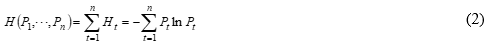where,.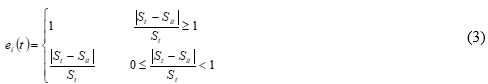where ei(t) is the relative error of the i-th detection method in the condition t, and {ei(t); i=1, 2, t=1,2,,20} is the relative error sequence of the i-th detection method under working condition t.

The relative error sequences of each detection methods are normalized to calculate the proportion of the relative error of the i-th detection method in the working condition t.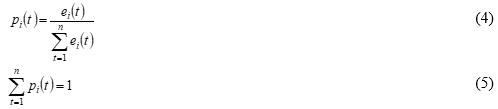The entropy value of the relative error of the i-th detection method is calculated as follows,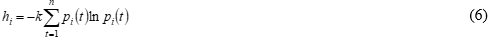where (k>0) is a constant. For the i-th detection method, if pi(t) are all equal (pi(t)=1/n), hi is going to be the maximum (hi=klnn), where k is taken 1/lnn, so there is 0≤h≤1.

From the definition of information entropy, it can be concluded that the smaller the entropy of a detection error sequence of a single detection method is, the greater its variation is (Lianxiao and Morimoto 2019).

Correlation coefficient

In multivariate analysis, the correlation coefficient is an indicator to measure the direction and degree of linear correlation between two random variables. The closer the detection value is to the actual value, the higher the correlation degree of the two sequences is. Therefore, correlation coefficient can be used to measure the detection precision. In this paper, the correlation coefficient (ri) between the detection sequence (Sit) and the actual sequence (St) is typically expressed as follows: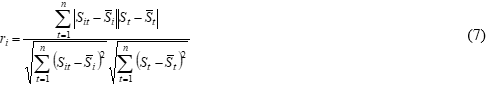Where,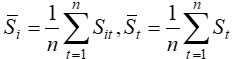.

(3) Residual sum of squares

In order to clearly explain what is the effect of each variate and random error. In statistics, the residual is the difference between the data point and its corresponding position on the regression line. The residual sum of squares represents the effect of random errors. The smaller the residual sum of squares is, the better the fitting degree is. In this paper, the square error sum (Qi) is taken as an indicator to reflect the detection precision, Qi can be defined as follows: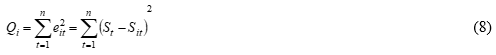Theory of fuzzy soft set and Bayesian formula

Molodtsov (1999) proposed the soft set theory. At present, soft set theory has become a general mathematical tool for dealing with uncertain and fuzzy objects. It is often used in decision-making, evaluation, parameter reduction, index weight determination, soft algebra, and prediction (Kovkov et al. 2007; Yue et al. 2013).

Definition 1 (soft set): Suppose that U is the initial discourse domain and is the parameter set, P (U) is the power set of U, (FL) is a soft set of U, if and only if F is a mapping from L to P (U). Each F(l) (lL) can be regarded as the set of lelements in the soft set (FL).

Definition 2 (fuzzy soft set): Suppose that U is the initial discourse domain and is the parameter set, ε(U) is the fuzzy set defined on U. (FA) (A ⊆ L) is a fuzzy soft set on the discourse domain U, if and only if F is a mapping from A to ε(U).

Definition 3 (Bayesian formula): Suppose that is the sample space of test E, and A is the event of E, (B1,B2,…,Bn) is a division of S, and P(A)>0, P(Bi)>0 (i=1,2,,n), then the Bayesian formula can be expressed as follows: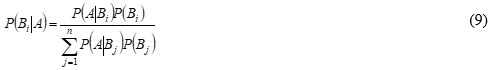To sum up, the steps to determine the combined weight coefficients based on fuzzy soft set and Bayesian method are as follows:

Step1: According to the detection results, the evaluation criteria (information entropy, correlation coefficient and residual sum of squares) for the detection results of two detection methods are calculated. Delphi method is used to perform fuzzy evaluation on the parameters of the evaluation criteria, and the fuzzy soft set (F, A) is input.

Step2: Input the initial discourse domain U.

Step3: Combining with the weights given by experts, the Bayesian formula (Eq.9) is used to modify the weights determined by the three evaluation criteria, respectively.

Step4: Calculate the average of the corrected weights.

EXPERIMENTAL

Non-Destructive Tests

Test Materials

Pine and elm, commonly used in the ancient wooden structures, were selected as test specimens. Through visual inspection, surface percussion, and pressing, there were no obvious joints, splits, or other damage on specimens. The test specimens were processed into round test pieces (Chang et al. 2016a; Wang et al. 2019). Table 1 shows the specific parameters. The average moisture content of the four specimens met the requirements of the GB/T 50005 (2017) and GB/T 50329 (2012) standards. Then, five standard circles are drawn on each specimen.

Table 1. Test Specimen Number and Parameters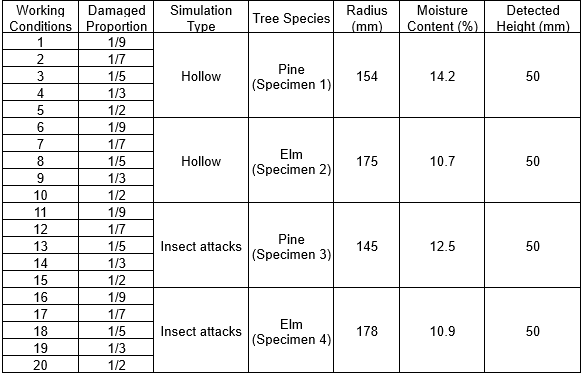The area of these five circular areas represented 1/9, 1/7, 1/5, 1/3 and 1/2 of the cross section area of each specimen, respectively (Fig. 3a). According to the idea of reverse simulation, the internal hollow and insect attacks were simulated by manual digging (Fig. 3b) and drilling (Fig. 3c) in the specimens. In addition, the diameter of the drilling is about 10 mm, and the distance between the drilling is about 5mm (Fig. 3c).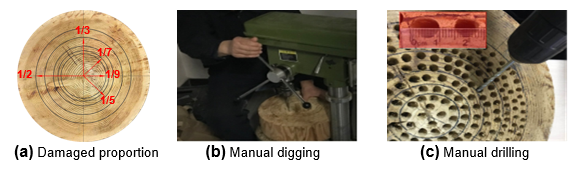Fig. 3. Making test specimens

Test conditions

The indoor temperature was 20 °C with 72% relative humidity. The test equipment (Fig. 4) was stress wave testing instrument (Fakopp, Enterprise, Hungary), drilling resistance testing instrument (IML-PD500, IML Co., Ltd., Wiesloch, Germany), wood moisture meter (GANN-HT85T, Gann Mess-u. Regeltechnik GmbH, Germany) and electric percussion drill (GSR18-2-LI, Robert Bosch GmbH, Stuttgart, Germany).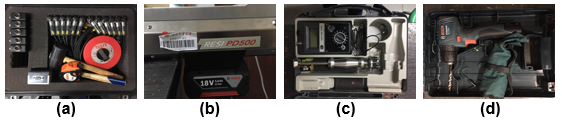Fig. 4. (a) Fakopp, (b) IML-PD500, (c) GANN-HT85T, (d) GSR18-2-LI

Stress Wave Detection

The Fakopp 3D acoustic tomograph (Fig. 5 and Fig. 6) is able to non-destructively detect the size and location of decayed or hollow parts in the trunk. It works based on sound velocity measurement between several sensors around the trunk. If there is a hole, the sound waves have to pass around the hole, and it requires more time to reach the opposite sensors. In order to explain the complex velocity model, a two-dimensional image is constructed. Healthy wood is shown in green, decaying wood is shown in red, and hollow is shown in blue. This test selects 10 sensors to detect the internal damage of the specimen. The damaged area (S1t) can be calculated according to detected damaged percentage which is obtained by stress wave ArborSonic 3D software.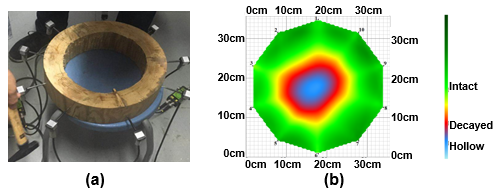Fig. 5. Stress wave detection for (a) hollow, (b) two-dimensional image of hollow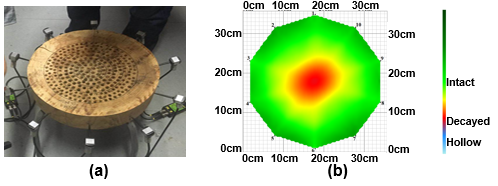Fig. 6. Stress wave detection for (a) Insect attacks, (b) two-dimensional image of Insect attacks

Drilling Resistance Detection

The IML-PD500 instrument (Fig. 7 and Fig. 8) uses a small needle, driven by a motor, to penetrate into the wood at a constant speed. The impedance curve is displayed on the display screen of the instrument. Drilling resistance detection is used to detect and judge the decay, hollow, and insect attacks of the wood. It can be used for the detection and evaluation of all trees and wooden structures. Because drilling resistance detection can only detect the damage of the wood in a single path, the test selects three paths to detect the specimen. The length of the internal damage measured for each path is d1d2 and d3. The damaged area (S2t) can be calculated by Eq. 10 (Chang et al. 2016a).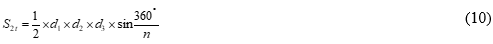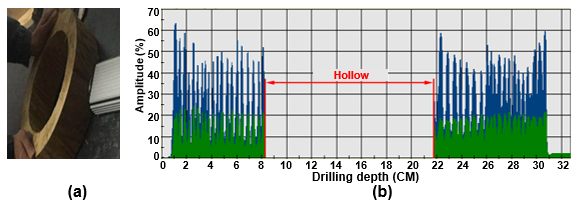Fig. 7. Drilling resistance detection for (a) hollow, (b) two-dimensional image of hollow